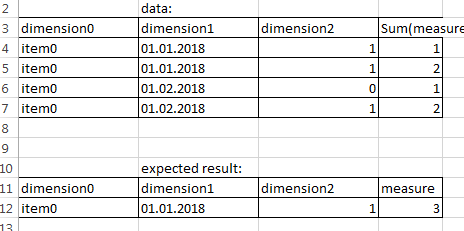# App Development

Announcements
cancel
Showing results for
Did you mean:Contributor

## exclude entire set if one item has predifined value

Hello!

Here is what i got:I have a list of items, dates and states. i want that if some dimension got state with some predefined value then all values got excluded.

So in example above dimension2 got 0 and i want to exclude everything(0, 2 and 1) because there is a zero in set.

Can i somehow achieve that? (via set analysis?)

1 Solution

Accepted SolutionsMVP

You might need to use Aggr() function here

Sum(Aggr(If(Count({<dimension2 = {0}>} dimension2) = 0, Sum(Measure)), dimension0, dimension1, dimension2))

4 RepliesMVP

You might need to use Aggr() function here

Sum(Aggr(If(Count({<dimension2 = {0}>} dimension2) = 0, Sum(Measure)), dimension0, dimension1, dimension2))MVP

Or maybe:

Sum(Aggr(If(Count(TOTAL <dimension0, dimension1> {<dimension2= {'0'}>} dimension2) = 0, Sum(measure)), dimension0, dimension1))Contributor
Author

Thanks! Thats exactly what i need.Contributor
Author